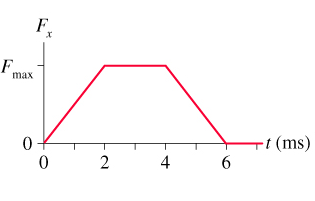# Problem: A 60g tennis ball with an initial speed of 32 m/s hits a wall and rebounds with the same speed. The figure shows the force of the wall on the ball during the collision. What is the value of Fmax, the maximum value of the contact force during the collision? (2 sig figs)

🤓 Based on our data, we think this question is relevant for Professor Arakelyan's class at BC.

###### FREE Expert Solution

Impulse:

$\overline{){\mathbf{I}}{\mathbf{=}}{\mathbf{F}}{\mathbf{t}}}$

Change in momentum:

$\overline{){\mathbf{∆}}{\mathbf{P}}{\mathbf{=}}{{\mathbf{mv}}}_{{\mathbf{f}}}{\mathbf{-}}{{\mathbf{mv}}}_{{\mathbf{0}}}}$

Impulse is equal to force under the curve:

I = (1/2)bh + l•w + (1/2)bh = (1/2)(2 - 0)Fmax + (4 - 2)Fmax + (1/2)(6 - 2)Fmax = 4Fmax N.ms

I = 4Fmax N.ms (10-3 s/ 1ms) = 0.004Fmax N•s

Change in momentum:###### Problem Details

A 60g tennis ball with an initial speed of 32 m/s hits a wall and rebounds with the same speed. The figure shows the force of the wall on the ball during the collision. What is the value of Fmax, the maximum value of the contact force during the collision? (2 sig figs)